# NCERT Solutions for Chapter 10 Tenths And Hundredths Class 5 Maths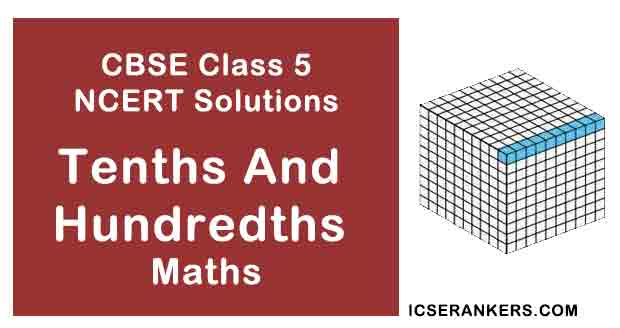Tenths And Hundredths Questions and Answers

 Chapter Name Tenths And Hundredths NCERT Solutions Class CBSE Class 5 Textbook Name Maths Related Readings NCERT Solutions for Class 5NCERT Solutions for Class 5 Maths Summary of Tenths And Hundredths

Page No 134:

Question 1: What was the length of the smallest pencil you have used?

How long is this pencil? Guess ________ cm Measure it using a scale.

How good is your guess? We can see that Anju used a lens to make it look bigger.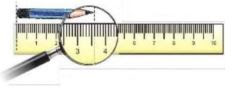The smallest pencil that I had used was about 1 cm long.

I think the given pencil is more than 3 cm.

With the help of scale, the length of the pencil comes out to be 3.6 cm. So, my guess was correct.

Disclaimer: The length of the smallest pencil that you have used varies from student to student. The answers provided here are for reference only.

Page No 135:

Question 1: What does 0.9 cm mean? It is the same as _______ millimetres. We can also say this is nine-tenths of a cm. Right? So 30.5 cm is the same as _______ cm and _______ millimeter. About how many of the big frogs will fit on the 1 m scale? _______ If they sit in a straight line about how many of the small frogs will cover 1 m? _______

We know that, 1 cm = 10 mm So, 0.9 cm = 9 mm 0.9 cm means 9 mm

It is the same as 9 millimetres.

So 30.5 cm is the same as 30 cm and 5 millimeter.

Now, 30.5 cm = 30 cm and 5 mm

We know that, 1 m = 100 cm

Length of the biggest frog = 30.5 cm Thus, a maximum of 3 big frogs can fit on 1m scale.

Length of each small frog = 0.9 cm Thus, a maximum of 111 small frogs can sit in a straight line and cover I m.

Question 2:

(1) Length of the nail-2 cm and _____ mm or 2. _____ cm.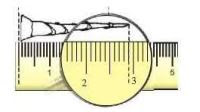(2)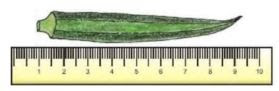The length of this lady finger (bhindi) is _____ cm and _____ mm. We can also write it as _____

cm.

(3) Using the scale on this page find the difference in length between candle 1 and candle 3.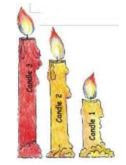Length of Length in cm and mm Length in cm Candle 1 Flame 1 Candle 2 Flame 2 Candle 3 Flame 3

(1) Length of nail = 2 cm 9 mm Now, 10 mm = 1 cm

1 mm = 110cm

9 mm = 0.9 cm

So, 2 cm 9 mm = 2.9 cm

(2) Length of lady finger is 8 cm and 4 mm Now, 10 mm = 1 cm

1 mm = 110cm

4 mm = 0.4 cm

So, 8 cm 4 mm = 8.4 cm

(3)

 Length of Length in cm and mm Length in cm Candle 1 2 cm 9 mm 2.9 cm Flame 1 1 cm 3 mm 1.3 cm Candle 2 4 cm 9 mm 4.9 cm Flame 2 1 cm 9 mm 1.9 cm Candle 3 6 cm 6.0 cm Flame 3 1 cm 9 mm 1.9 cm

Page No 137:

Question 1: Guess the lengths to draw these things. Ask your friend to draw the same. After you make the drawing use a scale to measure the length. Whose drawing showed a better guess?

 Guess it length and draw Measure of your drawing Measure of your friend’s drawing An ant of length less than 1 cm Pencil of length about 7 cm A glass 11 cm high with water up to 5 cm A bangle of perimeter 20 cm A curly hair of length 16 cm

Question 2: Which line is longer? A or B? Measure each line and write how long it is in centimetres. How good is your guess?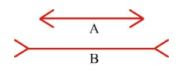Which line is longer? C or D? Measure each line. How good is your guess?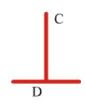I think line B is longer than line A. With the help of ruler, we get the measurement of line A as 4.6 cm and line B as 4.6 cm. That means, both the lines are of same length. So, our guess was incorrect.

I think both the lines C and D are of same measurement. With the help of ruler, we get the measurement as 3.2 cm for both the lines. So, our guess was correct.

Disclaimer: The guess may vary from student to student. The answers provided here are for reference only.

Page No 138:

Question 1: What is the length of a 100 rupee note? Guess. Now measure it using a scale.

Now guess the length and width of many other things. Measure and find the difference between your measure and your guess.

 Size of Your guess in cm Your measure in cm length width length width 100 Rupee note 10 Rupee note 20 Rupee note 5 Rupee note Post card Math-Magic book

My guess: Length of notebook = 25 cm Breadth of notebook = 20 cm.

Measure: Length of notebook = 22.3 cm Breadth of notebook = 18.3 cm

Difference in length between my guess and measure = 25 − 22.3 = 2.7 cm

Difference in breadth between my guess and measure = 20 − 18.3 = 1.7 cm

 Size of Your guess in cm Your measure in cm length width length width 100 Rupee note 10 Rupee note 20 Rupee note 5 Rupee note Post card Math-Magic book 20 15 12 10 12 25 10 7 6 5 7 20 15.8 13.7 14.7 11.7 10.5 24 7.3 6.3 6.3 6.4 6.5 18

Page No 139:

Question 1:

(1) How many paise does a matchbox cost? _____

(2) How many matchboxes can be got for Rs 2.50? _____

(3) How many rupees does the soap cost? _____

(4) Arun wanted to buy a soap. He has a five-rupee coin, 2 one-rupee coins and 4 half-rupee coins. Write in rupees what money he will get back.

(5) a) An egg costs two and a half rupees. How much will one and a half dozen cost? (b) How many pens can Kannan buy? How much money is left?

(6) The price of two pens is Rs _____ Can she buy two pens?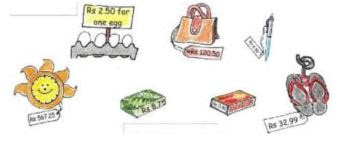We know that, Re 1 = 100 paise

(1) Cost of a matchbox = Re 0.50 = 50 paise

(2) Cost of a matchbox = Rs 50 paise Amount of money to buy matchboxes = Rs 2.50 = 2 rupees 50 paise = 2 × 100 paise + 50 paise = 200 paise + 50 paise = 250 paise

So, number of matchboxes bought for Rs 2.50 = 250 ÷ 50 = 5 Thus, 5 matchboxes can be bought for Rs 2.50.

(3) Cost of a soap = Rs 8.75

(4) We know that, Rs 1 = 100 paise  Arun has a five-rupee coin, 2 one- rupee coins and 4 half-rupee coins. Now, Rs 5 = 5 x 100 paise = 500 paise 2 one rupee coins = 2 × 100 paise = 200 paise 4 half-rupee coins = 4 × 50 paise = 200 paise Total money with Arun = 500 paise + 200 paise + 200 paise = 900 paise

Cost of a soap = Rs 8.75 = 8 rupees 75 paise = 8 x 100 paise + 75 paise = 800 paise + 75 paise = 875 paise

Money left with Arun after buying a soap = 900 paise – 875 paise = 25 paise = Rs 0.25

900 – 875 = 0.25 Thus, after buying a soap, Arun will get back Rs 0.25.

(5)

(a) Cost of an egg = Rs 2.50 = 2 rupees 50 paise = 2 × 100 paise + 50 paise 200 paise + 50 paise = 250 paise

Now, one dozen eggs = 12 eggs So, one and a half dozen eggs = 12 + 6= 18 eggs

Cost of 18 eggs = 18 × 250 paise = 4500 paise = Rs 45

250

X 18

2000 (250 x 8)

2500 (250 x 10)

4500

Thus, cost of one and half a dozen eggs is Rs 45.

(b) Total money with Kannan = Rs 60 Cost of one and a half dozen eggs = Rs 45 Total money left with Kannan after buying eggs = Rs 60 – 45 = Rs 15 = 1500 paise

Now, Kannan uses the money that is left for purchasing pens. Cost of a pen = Rs 6.50 = 6 rupees 50 paise = 6 x 100 paise + 50 paise = 600 paise + 50 paise = 650 paise

Cost of 2 pens = 2 × 650 paise = 1300 paise = Rs 13

So, Kannan can buy only two pens with the amount of money that was left with him after purchasing eggs. Now, money left with Kannan after purchasing pens 1500 paise 1300 paise 200 paise = Rs 2 Thus, Kannan was left with Rs 2 after buying 2 pens.

(6) The price of two pens is Rs 13. She can buy two pens.

Page No 140:

Question 1: Match each yellow box with one green and one pink box.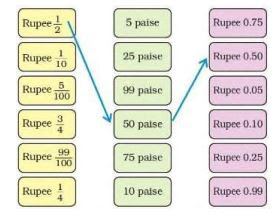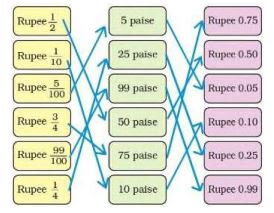Question 2: Colourful Design What part of this sheet is coloured blue? _____ /10 What part of the sheet is green? _____ Which colour covers 0.2 of the sheet?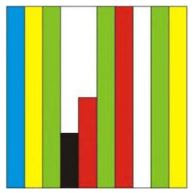Total number of strips in the sheet = 10

Number of blue coloured strips = 1

So, part of the sheet that is blue = 1/10

Thus, 1/10 of the sheet is blue.

Number of green coloured strips = 3

So, part of the sheet that is green = 3/10

Thus, 3/10 of the sheet is green.

Number of yellow coloured strips = 2

So, part of the sheet that is yellow = 2/10 = 0.2

Thus, 2/10 of the sheet is yellow.

Question 3: Look at the second sheet. Each strip is divided into 10 equal boxes. How many boxes are there in all?Is each box 1/100 part of the sheet? How many blue boxes are there?____ Is blue equal to 10/100 of the sheet? We saw that blue is also equal to 1/10 of the sheet. We wrote it as 0.1 of the sheet.

Can we say 10/100 = 1/10 = 0.10 = 0.1?

Think: Can we write ten paise as 0.1 of a rupee? How many boxes are red? What part of the sheet is this? 15/_____ Can we also write it as 0.15 of the sheet? Now 3/100 of the sheet is black. We can say 0. _____sheet is black. How many white boxes are there in the sheet? What part of the second sheet is white? _____

Number of boxes in 1 strip= 10 There are a total of 10 strips in the sheet. So, number of boxes in 10 strips = 10 x 10 = 100 Yes, each box is

1/100 part of the sheet.

There are 10 blue boxes in the sheet. Yes, blue equals to

10/100 of the sheet. It is given that blue is equal to

1/10 of the sheet. Now,

1/10 =1 ×10/ 10×10 = 10/100

Thus, 1/10 is also equal to

10/100 of the sheet. And,

1/10 = 0.1

So,

10/100 = 1/10 = 0.10 = 0.1

Now, 100 paise = Re 1 So, we need ten 10 paise coins to make Re 1. Thus, we can say that 10 paise is

1/10 of a rupee. Now,

1/10 = 0 .1 So, 10 paise is s 0.1 of a rupee.

There are a total of 15 red boxes in the sheet.

Total number of boxes in the sheet = 100 Part of the sheet that is red = 15/100

Now,

15/100 = 0.15 So, 0.15 of the sheet is red.

It is given that 3/100 of the sheet is black. Now,

3/100 = 0.03 So, we can say that 0.03 of the sheet is black.

There are a total of 22 white boxes in the sheet.

So, part of the sheet that is white =

22/100 = 0.22 Thus, 0.22 of the sheet is white.

Page No 141:

Question 1: The school at Malappuram has its sports day. The first five children in the Long Jump are: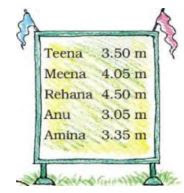Who is the winner in the long jump? __________ Write the names of the I, II and III winners on this stand.

 Do you remember that 1 metre = 100 centimetre?  So one centimetre is 1/100 of a metre. We also write 1 cm as _____ m

It is given that 4.50 m is longest jump.
Thus, Rehana is the winner in the long jump.
The 1ST winner is Rehana, 2nd winner is Meena, and 3rd winner is Teena.
We know that, 100 cm = 1 m so, 1 cm =
1/100 m 1 cm =  0.01 m
So, we can also write 1 cm as 0.01 m.

Page No 142:

Question 1: Write in Metres

3 metre 45 centimetre

99 centimetre

1 metre and 5 centimetre

We know that, 100 cm = 1 m 1 cm =

1/100 m = 0.01 m Now, 3 m 45 cm = 3 + 45/100 m =3 + 0.45 m = 3.45 m

99 cm = 99/100 m = 0.99 m

1m 5 cm = 1 + 5/100 m =1 + 0.05 m = 1.05 m

3 metre 45 centimetre                    3.45 metres

99 centimetre                                 0.99 metres

I metre and 5 centimetre                1.05 metres

Question 2: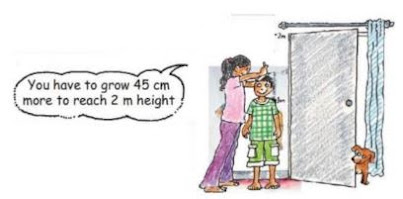What is Dinesh's height in metres? _____ m_____ cm.

Dinesh has to grow 45 cm more to reach the height of 2 m. Now, 1 m =

100 cm So, 2 m = 200 cm

Height of Dinesh = 200 − 45 = 155 cm

200 – 45 =155 Now, 155 cm = 100 + 55 cm = 1 m 55 cm

Thus, Dinesh's height is 1 m 55 cm.

Page No 143:

Question 1: Shivam Bank has a chart to show us how many Indian rupees we can get when we change the money of different countries.

 Country Money Changed into Indian Rupees Korea Sri Lanka Nepal Hong Kong South Africa                China U.A.E. U.S.A. Germany England Won Rupee(SL) Rupee Dollar(HK)                Rand Yuan Dirham Dollar Euro Pound 0.04 0.37 0.63 5.10 5.18 5.50 10.80 39.70 58.30 77.76
(This is the rate on 15-2-2008)

(A) The money of which country will cost the most in Indian Rupees?

(B) Mithun's uncle in America had sent him 10 USA dollars as a gift. Mithun used 350 rupees for a school trip. How much money was left with him?

(C) Majeed's father is working in Saudi Arabia. He gets 1000 Saudi Riyal as salary. Arun's father who is working in Sri Lanka gets 2000 Sri Lankan Rupees. Who gets more Indian rupees as salary?

(D) Leena's aunty brought a present for her from China. It cost 30 Yuan. Find what it costs in Indian rupees.

(E) Astha wants some Hong Kong Dollars and Won.

(1) How many Won can she change for Rs 4? For Rs 400?

(2) How many Hong Kong Dollars can she change for Rs 508?

(A) The money of England will cost the most in Indian Rupees as I pound is equal to Rs 77.76

(B) We find from the chart that, I US dollars = Rs 39.70 = 39 rupees 70 paise = 39 × 100 paise + 70 paise = 3900 paise + 70 paise = 3970 paise 10 US dollars = 10 × 3970 paise = 39700 paise = Rs 397.00

So, Mithun got Rs 397.00 from his uncle as a gift.

Money spent on school trip by Mithun = Rs 350.00 So, money left with Mithun = Rs 397.00 − 350.00 = Rs 47.00

(C) Here, we are considering the exchange rates of Dirham in place of Saudi Riyal. Salary of Majeed's father = 1000 Dirham

We find from the chart that, I Dirham Rs 10.80 = 10 rupees 80 paise = 10 ×100+ 80 paise = 1000 + 80 paise = 1080 paise

So, 1000 Dirhams = 1000 × 1080 paise = 1080000 paise = Rs 10800

Therefore, salary of Majeed's father= Rs 10800.00

Salary of Arun's father= 2000 Sri Lankan Rupees We find from the chart that, 1 Sri Lankan Rupee = Re 0.37 = 37 paise

So, 2000 Sri Lankan Rupees = 2000 × 37 paise = 74000 paise = Rs 740.00

Therefore, salary of Arun's father= Rs 740

Thus, Majeed's father gets more salary in Indian Rupees than Arun's father.

(D) Price of present bought by Leena's aunty = 30 Yuan

We find from the chart that,

1 Yuan = Rs 5.50 = 5 rupees 50 paise = 5 â€‹ × 100 + 50 paise = 500+50 paise = 550 paise

So, 30 Yuan = 30 â€‹ × 550 paise = 16500 paise = Rs 165

So, the cost of the gift in Indian Rupees is Rs 165.00

(E)

(1) We find from the chart,

1 Won = Re 0.04 = 4 paise

We know that, Re 1 = 100 paise So, Rs 4 = 4  × 100 paise = 400

paise Rs 400 = 400 × 100 paise = 40000 paise

Now, 4 paise = I Won So, 400 paise = 400 ÷ 4 Won = 100 Won and 40000 paise = 40000 â€‹÷ 4             Won

= 10000 Won

Thus, Astha can change 100 Won for Rs 4, and 10000 Won for Rs 400.

(2) We find from the chart,

1 Hong Kong Dollar = Rs 5.10 = 5 rupees 10 paise = 5 × 100 + 10 paise = 500 + 10 paise = 510            paise

Total money with Astha = Rs 508 = 508 × 100 paise = 50800 paise

50800 paise = 50490 + 310 paise

50800 paise = 510 × 99 + 310 paise

50800 paise = 510 HKD + 310 paise

Now, 310 paise = 300 paise + 10 paise = 3 rupees and 10 paise = Rs 3.10

Thus, Astha can change 99 HKD for Rs 508 and Rs 3.10 is left with her.

Page No 144:

Question 1: Kiran went shopping with Rs 200. Look at the bill. The shopkeeper forgot to put the point correctly in the prices. Put the point in the correct place and find out the total amount of the bill.

 Item Quantity Price(Rupees) Soap  Green  Gram tea  Coconut oil 1  1 kg  250 gm     1 litre 1250  5025  2725  6000 Total _____

 Item Quantity Price(Rupees) Soap  Green  Gram tea  Coconut oil 1  1 kg  250 gm     1 litre 12.50  50.25  27.25  60.00 Total 150.00

Page No 145:

Question 1: The temperature in each city was noted at 3 pm on 16 January 2008.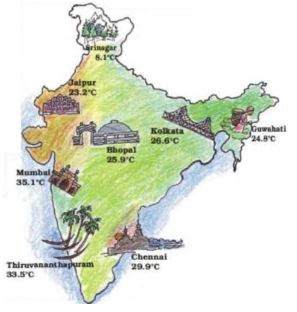(1) Which place had the highest temperature at 3 pm? Which place is the coolest at that time?

(2) How much higher is the temperature in Mumbai from that in Srinagar?

(3) How many degrees will the temperature need to rise for it to reach 40°C in Thiruvananthapuram?

(4) How much lower is the temperature of Kolkata from that in Chennai?

(5) The temperature in these cities was also noted at 3 am on the same day. Look at the table and answer the questions.

(a) Which place had the lowest temperature at 3 am? Imagine yourself to be there and
describe how it would feel.

(b) What is the difference between the temperatures at 3 pm and 3 am in Chennai? In Bhopal?

 City Temperature at 3 am Chennai 21.1 Mumbai 19.0 Thiruvananthapuram 21.6 Kolkata 13.1 Bhopal 9.8 shrinagar 1.3 Guwahati 12.8 Jaipur 10.2

(1) Mumbai had the highest temperature i.e. 35.1°C at 3 pm.
Srinagar was the coolest place with the temperature of 8.1°C at 3 pm.

(2) Temperature of Mumbai at 3 pm = 35.1°C
Temperature of Srinagar at 3 pm = 8.1°C
Difference in temperature 35.1° C − 8.1° C = 27°C

(3) Temperature of Thiruvananthapuram at 3 pm = 33. 5° C
Rise in the temperature needed to attain a temperature of 40°C = 40 °C − 33.5°C = 6.5° C

(4) Temperature of Chennai at 3 pm = 29.9°C
Temperature of Kolkata at 3 pm = 26.6°C
Difference between  temperature 29.9°C− 26.6° C = 3.3°C

(5)

(a) Temperature of Srinagar at 3 am = 1.3°C It will be very cold there.

(b) Temperature of Chennai at 3 pm = 29.9°C
Temperature of Chennai at 3 am = 21.1°C
Difference between temperature = 29.9°C − 21.1 °C= 8.8 °C

Temperature of Bhopal at 3 pm = 25.9°C
Temperature of Bhopal at 3 am = 9.8°C
Difference between temperature = 25.9°C − 9.8°C = 16.1° C